# Witt vector

(diff) ← Older revision | Latest revision (diff) | Newer revision → (diff)

An element of an algebraic construct, first proposed by E. Witt  in 1936 in the context of the description of unramified extensions of-adic number fields. Witt vectors were subsequently utilized in the study of algebraic varieties over a field of positive characteristic , in the theory of commutative algebraic groups , , and in the theory of formal groups . Letbe an associative, commutative ring with unit element. Witt vectors with components inare infinite sequences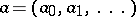,, which are added and multiplied in accordance with the following rules: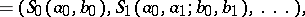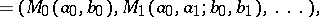where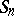,are polynomials in the variables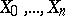,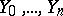with integer coefficients, uniquely defined by the conditions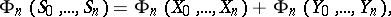where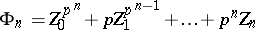are polynomials,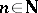andis a prime number. In particular,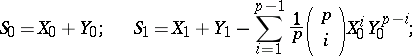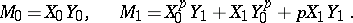The Witt vectors with the operations introduced above form a ring, called the ring of Witt vectors and denoted by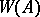. For any natural numberthere also exists a definition of the ringof truncated Witt vectors of length. The elements of this ring are finite tuples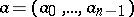,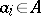, with the addition and multiplication operations described above. The canonical mappings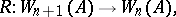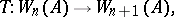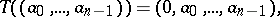are homomorphisms. The rule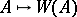(or) defines a covariant functor from the category of commutative rings with unit element into the category of rings. This functor may be represented by the ring of polynomials(or) on which the structure of a ring object has been defined. The spectrum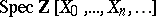(or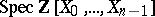) is known as a Witt scheme (or a truncated Witt scheme) and is a ring scheme .

Each elementdefines a Witt vector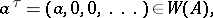called the Teichmüller representative of the element. If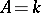is a perfect field of characteristic,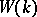is a complete discrete valuation ring of zero characteristic with field of residuesand maximal ideal. Each element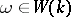can be uniquely represented as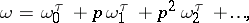where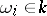. Conversely, each such ringwith field of residues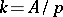is canonically isomorphic to the ring. The Teichmüller representation makes it possible to construct a canonical multiplicative homomorphism, splitting the mapping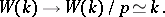If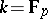is the prime field ofelements,is the ring of integral-adic numbers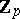.